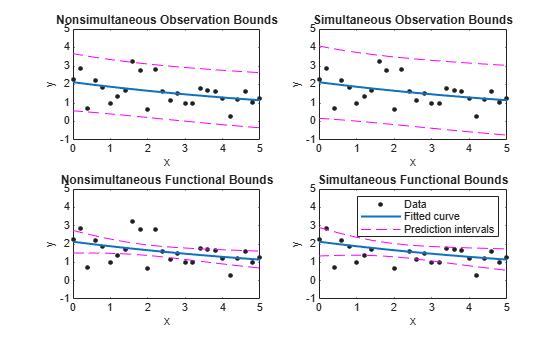# predint

Prediction intervals for `cfit` or `sfit` object

## Syntax

```ci = predint(fitresult,x) ci = predint(fitresult,x,level) ci = predint(fitresult,x,level,intopt,simopt) [ci,y] = predint(...) ```

## Description

`ci = predint(fitresult,x)` returns upper and lower 95% prediction bounds for response values associated with the `cfit` object `fitresult` at the new predictor values specified by the vector `x`. `fitresult` must be an output from the `fit` function to contain the necessary information for `ci`. `ci` is an `n`-by-2 array where `n = length(x)`. The left column of `ci` contains the lower bound for each coefficient; the right column contains the upper bound.

`ci = predint(fitresult,x,level)` returns prediction bounds with a confidence level specified by `level`. `level` must be between `0` and `1`. The default value of `level` is `0.95`.

`ci = predint(fitresult,x,level,intopt,simopt)` specifies the type of bounds to compute.

`intopt` is one of

• `'observation'` — Bounds for a new observation (default)

• `'functional'` — Bounds for the fitted curve

`simopt` is one of

• `'off'` — Non-simultaneous bounds (default)

• `'on'` — Simultaneous bounds

Observation bounds are wider than functional bounds because they measure the uncertainty of predicting the fitted curve plus the random variation in the new observation. Non-simultaneous bounds are for individual elements of `x`; simultaneous bounds are for all elements of `x`.

`[ci,y] = predint(...)` returns the response values `y` predicted by `fitresult` at the predictors in `x`.

### Note

`predint` cannot compute prediction intervals for non-parametric regression methods such as `Interpolant`, `Lowess`, and `Spline`.

## Examples

collapse all

Compute and plot observation and functional prediction intervals for a fit to noisy data.

Generate noisy data with an exponential trend.

```x = (0:0.2:5)'; y = 2*exp(-0.2*x) + 0.5*randn(size(x));```

Fit a curve to the data using a single-term exponential.

`fitresult = fit(x,y,'exp1');`

Compute 95% observation and functional prediction intervals, both simultaneous and nonsimultaneous.

```p11 = predint(fitresult,x,0.95,'observation','off'); p12 = predint(fitresult,x,0.95,'observation','on'); p21 = predint(fitresult,x,0.95,'functional','off'); p22 = predint(fitresult,x,0.95,'functional','on');```

Plot the data, fit, and prediction intervals. Note that the observation bounds are wider than the functional bounds.

```subplot(2,2,1) plot(fitresult,x,y), hold on, plot(x,p11,'m--'), xlim([0 5]), ylim([-1 5]) title('Nonsimultaneous Observation Bounds','FontSize',9) legend off subplot(2,2,2) plot(fitresult,x,y), hold on, plot(x,p12,'m--'), xlim([0 5]), ylim([-1 5]) title('Simultaneous Observation Bounds','FontSize',9) legend off subplot(2,2,3) plot(fitresult,x,y), hold on, plot(x,p21,'m--'), xlim([0 5]), ylim([-1 5]) title('Nonsimultaneous Functional Bounds','FontSize',9) legend off subplot(2,2,4) plot(fitresult,x,y), hold on, plot(x,p22,'m--'), xlim([0 5]), ylim([-1 5]) title('Simultaneous Functional Bounds','FontSize',9) legend({'Data','Fitted curve', 'Prediction intervals'},... 'FontSize',8,'Location','northeast')```